# Moments (Part - 1) - Forces and Moments Civil Engineering (CE) Notes | EduRev

## Civil Engineering (CE) : Moments (Part - 1) - Forces and Moments Civil Engineering (CE) Notes | EduRev

The document Moments (Part - 1) - Forces and Moments Civil Engineering (CE) Notes | EduRev is a part of the Civil Engineering (CE) Course Introduction to Dynamics and Vibrations- Notes, Videos, MCQs.
All you need of Civil Engineering (CE) at this link: Civil Engineering (CE)

The moment of a force is a measure of its tendency to rotate an object about some point. The physical significance of a moment will be discussed later. We begin by stating the mathematical definition of the moment of a force about a point.

2.2.1 Definition of the moment of a force.

To calculate the moment of a force about some point, we need to know three things:

1. The force vector, expressed as components in a basis (Fx, Fy, Fz), or better as F = Fxi + Fyj + Fzk

2. The position vector (relative to some convenient origin) of the point where the force is acting (x, y, z) or better r = xi +yj +zk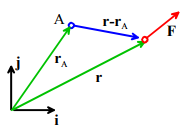3. The position vector of the point (say point A) we wish to take moments about (you must use the same origin as for 2) (x (A), y(A) ,z(A)) or rA =  x(A)i+y (A) j+z(A)

The moment of F about point A is then defined as

MA = (r − rA) × F

We can write out the formula for the components of MA in longhand by using the definition of a cross product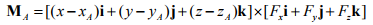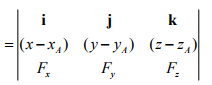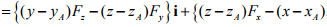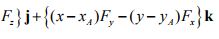The moment of F about the origin is a bit simpler

M0 = r × F

or, in terms of components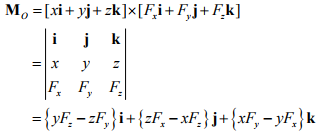2.2.2 Resultant moment exerted by a force system.

Suppose that N forces F(1)F(2) .....F(N) act at positions r(1), r(2),....r(N). The resultant moment of the force system is simply the sum of the moments exerted by the forces. You can calculate the resultant moment by first calculating the moment of each force, and then adding all the moments together (using vector sums).

Just one word of caution is in order here – when you compute the resultant moment, you must take moments about the same point for every force.

Taking moments about a different point for each force and adding the result is meaningless!

2.2.3 Examples of moment calculations using the vector formulas

We work through a few examples of moment calculations

Example 1: The beam shown below is uniform and has weight W. Calculate the moment exerted by the gravitational force about points A and B.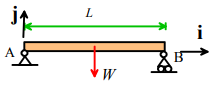We know (from the table provided earlier) that the center of gravity is half-way along the beam. The force (as a vector) is

F = Wj

To calculate the moment about A, we take the origin at A. The position vector of the force relative to A is

r = (L/2)i

MA = r× F = (L / 2)i × (−W)j = −(WL / 2)k

To calculate the moment about B, we take B as the origin. The position vector of the force relative to B is

r = −( L/ 2)i

Therefore

MB =r× F = (−L / 2)i × (−W )j = (WL / 2)k

Example 2. Member AB of a roof-truss is subjected to a vertical gravitational force W and a horizontal wind load P.  Calculate the moment of the resultant force about B.

r = (− L / 2)i − (L / 2) tanθ j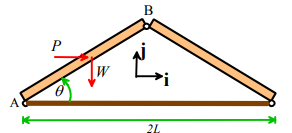The resultant force is

F  = Pi Wj

Therefore the moment about B is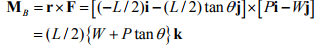Example 3. The structure shown is subjected to a force T acting at E along the line EF. Calculate the moment of T about points A and D.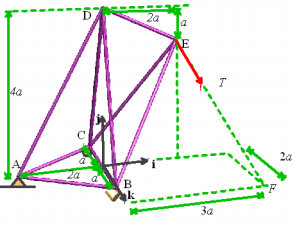This example requires a lot more work. First we need to write down the force as a vector. We know the magnitude of the force is T, so we only need to work out its direction. Since the force acts along EF, the direction must be a unit vector pointing along EF. It’s not hard to see that the vector EF is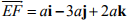We can divide by the length of EF (a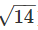) to find a unit vector pointing in the correct direction

eEF = (i -3j +2k) /The force vector is

F = T(i -3j +2k) /Next, we need to write down the necessary position vectors

Force: r = 2ai+3aj
Point A: rA = -2ai
Point D: rD = 4aj

Finally, we can work through the necessary cross products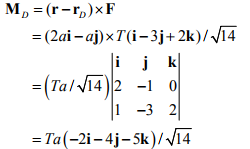Clearly, vector notation is very helpful when solving 3D problems!

Example 4. Finally, we work through a simple problem involving distributed loading. Calculate expressions for the moments exerted by the pressure acting on the beam about points A and B.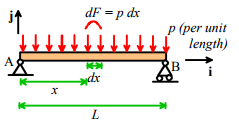An arbitrary strip of the beam with length dx is subjected to a force

dF = -pdx j

The position vector of the strip relative to A is

r = xi

The force acting on the strip therefore exerts a moment

dMA =xi × (− p dx) j = − px dx k

The total moment follows by summing (integrating) the forces over the entire length of the beam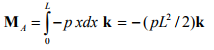The position vector of the strip relative to B is

r = (L = x)i

The force acting on the strip exerts a moment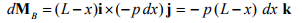The total moment follows by summing (integrating) the forces over the entire length of the beam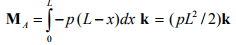2.2.4 The Physical Significance of a Moment

A force acting on a solid object has two effects: (i) it tends to accelerate the object (making the object’s center of mass move); and (ii) it tends to cause the object to rotate

1. The moment of a force about some point quantifies its tendency to rotate an object about that point.

2. The magnitude of the moment specifies the magnitude of the rotational force.

3. The direction of a moment specifies the axis of rotation associated with the rotational force, following the right hand screw convention.

Let’s explore these statements in more detail.

The best way to understand the physical significance of a moment is to think about the simple experiments you did with levers & weights back in kindergarten. Consider a beam that’s pivoted about some point (e.g. a see-saw).

Hang a weight W at some distance d to the left of the pivot, and the beam will rotate (counter-clockwise)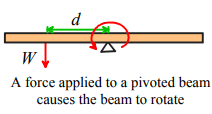To stop the beam rotating, we need to hang a weight on the right side of the pivot. We could

(a) Hang a weight W a distance d to the right of the pivot
(b) Hang a weight 2W a distance d/2 to the right of the pivot
(c) Hang a weight W/2 a distance 2d to the right of the pivot
(d) Hang a weight αW a distance d /α to the right of the pivot.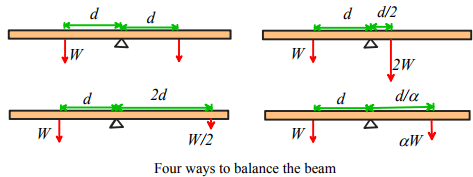These simple experiments suggest that the turning tendency of a force about some point is equal to the distance from the point multiplied by the force. This is certainly consistent with MO = r × F

To see where the cross product in the definition comes from, we need to do a rather more sophisticated experiment. Let’s now apply a force F at a distance d from the pivot, but now instead of making the force act perpendicular to the pivot, let’s make it act at some angle. Does this have a turning tendency Fd?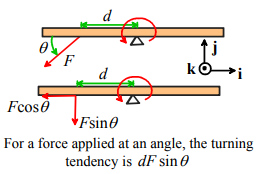A little reflection shows that this cannot be the case. The force F can be split into two components – F sinθ perpendicular to the beam, and F cosθ parallel to it. But the component parallel to the beam will not tend to turn the beam. The turning tendency is only dF sinθ .

Let’s compare this with MO = r × F Take the origin at the pivot, then

r =−di F =−F cosθi − F sinθj ⇒ r ×F = dF sinθk

so the magnitude of the moment correctly gives the magnitude of the turning tendency of the force. That’s why the definition of a moment needs a cross product.

Finally we need to think about the significance of the direction of the moment. We can get some insight by calculating MO = r × F for forces acting on our beam to the right and left of the pivot

For the force acting on the left of the pivot, we just found

r =−di F =−F cosθi − F sinθj ⇒ r ×F = dF sinθk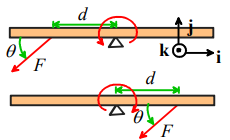For the force acting on the right of the pivot

r = di F= −F cosθi − F sinθj ⇒ r ×F = −dF sinθk

Thus, the force on the left exerts a moment along the +k direction, while the force on the right exerts a moment in the –k direction.

Notice also that the force on the left causes counterclockwise rotation; the force on the right causes clockwise rotation. Clearly, the direction of the moment has something to do with the direction of the turning tendency.

Specifically, the direction of a moment specifies the axis associated with the rotational force, following the right hand screw convention.

It’s best to use the screw rule to visualize the effect of a moment – hold your right hand as shown, with the thumb pointing along the direction of the moment. Your curling fingers (moving from your palm to the finger tips) then indicate the rotational tendency associated with the moment. Try this for the beam problem. With your thumb pointing along +k (out of the picture), your fingers curl counterclockwise. With your thumb pointing along –k, your fingers curl clockwise.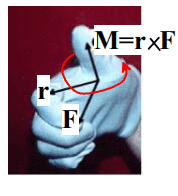Offer running on EduRev: Apply code STAYHOME200 to get INR 200 off on our premium plan EduRev Infinity!

## Introduction to Dynamics and Vibrations- Notes, Videos, MCQs

20 videos|53 docs

,

,

,

,

,

,

,

,

,

,

,

,

,

,

,

,

,

,

,

,

,

;